# Benchmark Class

The "benchmark class" is the class of prediction strategies in competitive on-line prediction which a given prediction algorithm is designed to compete with. The algorithm competes successfully with the class if its cumulative loss over the first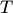trials of the game does not exceed, or is approximately equal to, the loss of the best strategies in the class (or, for "big" benchmark classes, to the loss of the best strategies whose "complexity", such as the norm, is not too large). Usually one compares the losses by inequalities, bounded the difference between the loss of the algorithm and the loss of the best strategy, named Regret term. The order of the regret terms presented in this article is for the mixable loss functions.

• In the case of prediction with expert advice (in the narrow sense of this wiki), the benchmark class is the finite set of experts, also called the pool of experts. The regret term usually depends on the number of experts as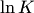, and does not depend on.
• It is usually possible to extend the algorithms for the class described above to the case of a countably infinite number of experts. In this case the regret term is of the order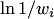, where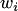is a weight initially assigned to expert.
• The next step is to compete with the "switching" experts, or "superexperts" who repeat the predictions of a chosen expert but sometimes switch to another expert (say, with a given probability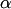). The Tracking the Best Expert algorithm was developed to compete with such type of predictors. The regret term in this case depends on the number of switches too.
• One can compete with all possible linear combinations of experts, which is equivalent to the problem of online linear regression. In this case there is some input vector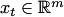, and the benchmark class is the set of all possible vectors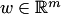. The algorithm is competing with the linear functions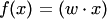. The regret term is of order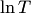.
• A wider class, often considered in literature, is the class of functions from some bounded subset of a reproducing kernel Hilbert space. The kernel trick used in algorithms to compete with such spaces provides their computational efficiency. The regret term depends onas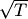.
• One can compete with the class of functions from the Besov spaces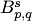satisfying the condition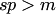(see "Competing with Besov spaces" for the importance of this condition). The important parameters are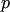and;is responsible for the rotundity of the unit ball, andfor the smoothness of functions in the space. The two techniques that have been applied to these benchmark classes (Defensive Forecasting and Metric Entropy) lead to results that are not comparable. This gives rise to an open problem: see Competing with Besov spaces.